﻿

### A remark about the embedding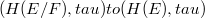$(H(E/F),tau)to(H(E),tau)$, with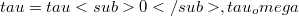$tau=tau0,tau_omega$, in Frechet spaces

#### Abstract

In a recentpaper by Aron-Moraes-Ryan , it is proved that when E is a complex Banach space, F is a closed subspace of E and U is a balanced open subset of E, then the mapping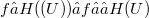$f ∈ H(𝜋(U)) → f ○ 𝜋 ∈ H(U)$ where 𝜋 is the canonical mapping from E onto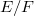$E/F$, is a topological isomorphism from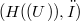$(H(𝜋(U)),τ)$ onto a closed subspace of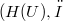$(H(U),τ$, where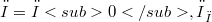$τ = τ0,τ_ω$. The aim of this remark is to show that the same result is true, with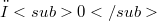$τ0$ for Fréchet spaces, and with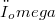$τ_omega$ for Fréchet-Schwartz spaces. Also we prove that this result is not true, with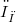$τ_ω$ for some Fréchet-Montel spaces and with$τ_δ$ for some nuclear Fréchet spaces.

DOI Code: 10.1285/i15900932v9n2p217

Full Text: PDF